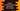# Python find the base name of a given path## How to find the base name of a given path in python:

os module provides different methods for operating system related tasks. os.path provides different methods for pathname manipulation. basename() is a method defined in os.path. basename() method is used to find the base name of a given path. This method is not same as the unix basename and output might vary.

os.path.basename() method takes the full path as the parameter and returns the base name of the path.

### Definition of os.path.basename:

os.path.basename is defined as below:

``os.path.basename(path)``

This method uses split() method to get the base name.

### Example of os.path.basename:

Let’s take a look at the program below:

``````import os

path1 = '/user/cvc/documents'
print('path1 = {}'.format(os.path.basename(path1)))

path2 = '/user/cvc/documents/'
print('path2 = {}'.format(os.path.basename(path2)))

path3 = '/user/cvc/documents/file.png'
print('path3 = {}'.format(os.path.basename(path3)))

path4 = 'file.png'
print('path4 = {}'.format(os.path.basename(path4)))``````

It will print the below output:

``````path1 = documents
path2 =
path3 = file.png
path4 = file.png``````